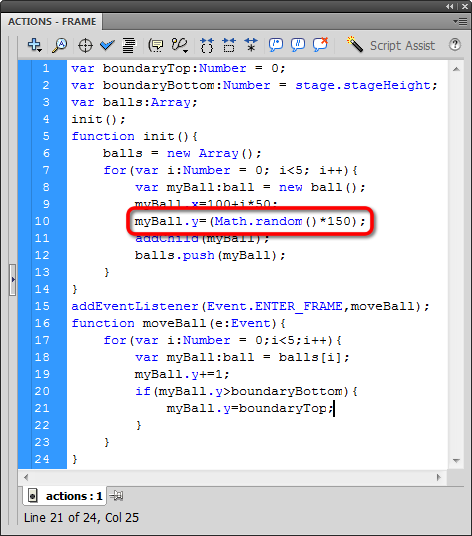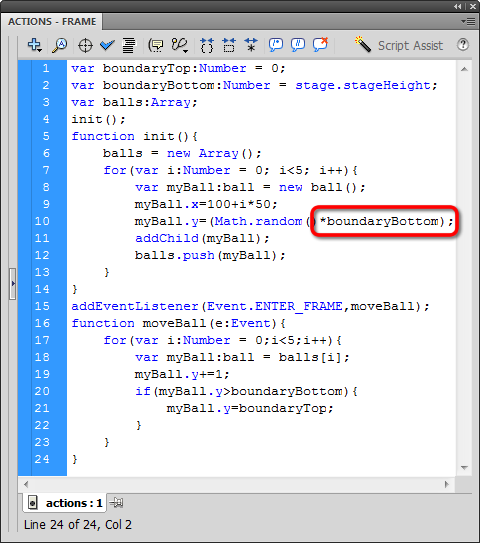# Wrap Multiple Objects

## random numbers

Finally, we want to create a vertical spread in our init function, so those balls aren't all just moving in unison. We will randomize the spread by having ActionScript generate a random number using the built-in Math.random function:

Math.random()*number;

Replace number with the value you want to randomize. Let's choose 50:

Math.random()*50;

ActionScript will return one of 50 possible values, from 0 to 49. That's not a typo, ActionScript will never return the number 50, because it starts counting at 0.

Let's put this in action. Set the Y position of myBall to be a random number between 0 and 149:Remember, we're randomizing as part of the init function. If that statement in the moveBall function, you'll have balls jumping all over the screen! Now test it:

Of course we can use Math.random with a variable! Let's set the Y position to be a random number anywhere within our boundaries. We've already created a boundaryBottom variable, so we can use that:Hooray for variables! Check it out: